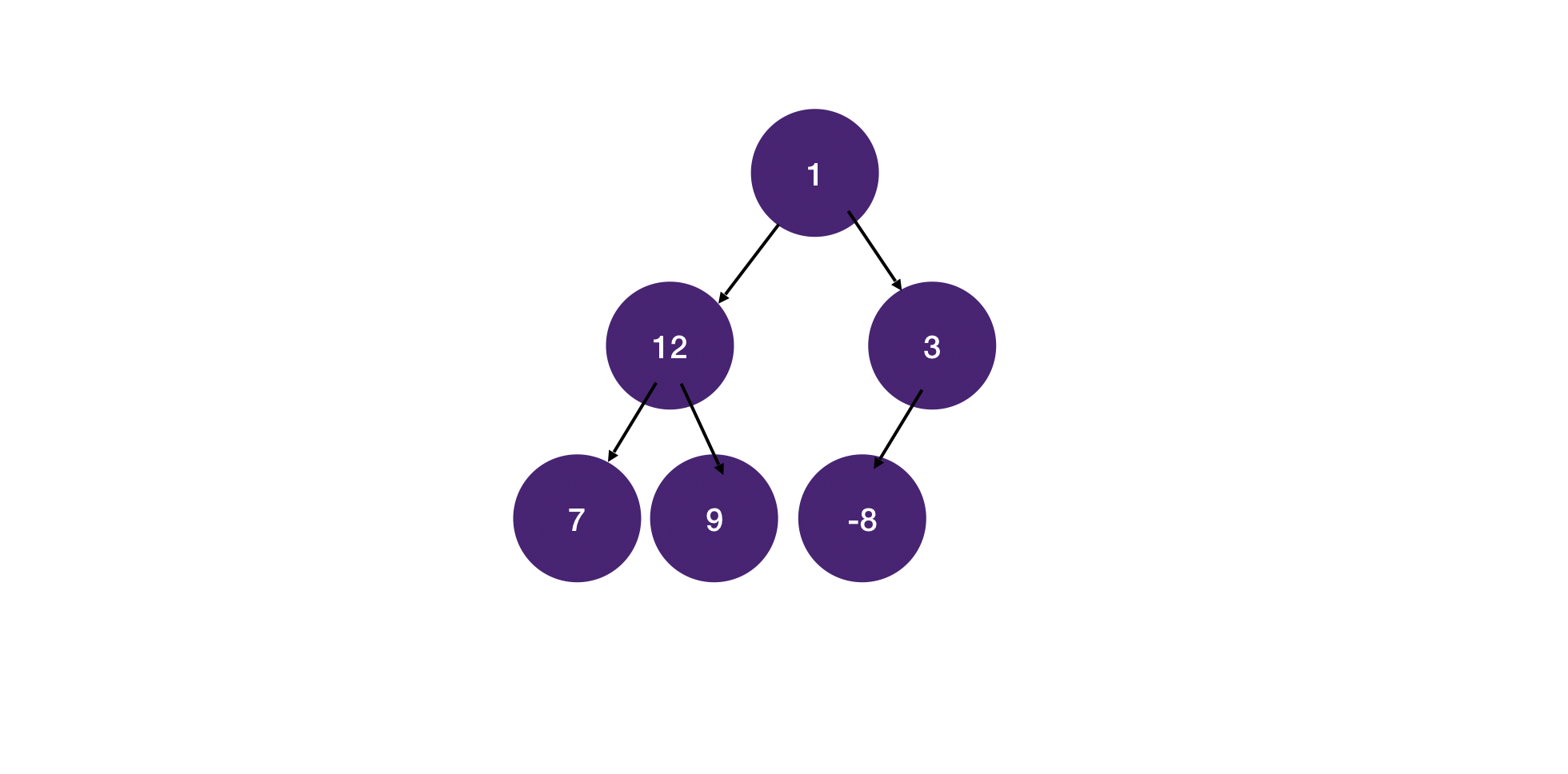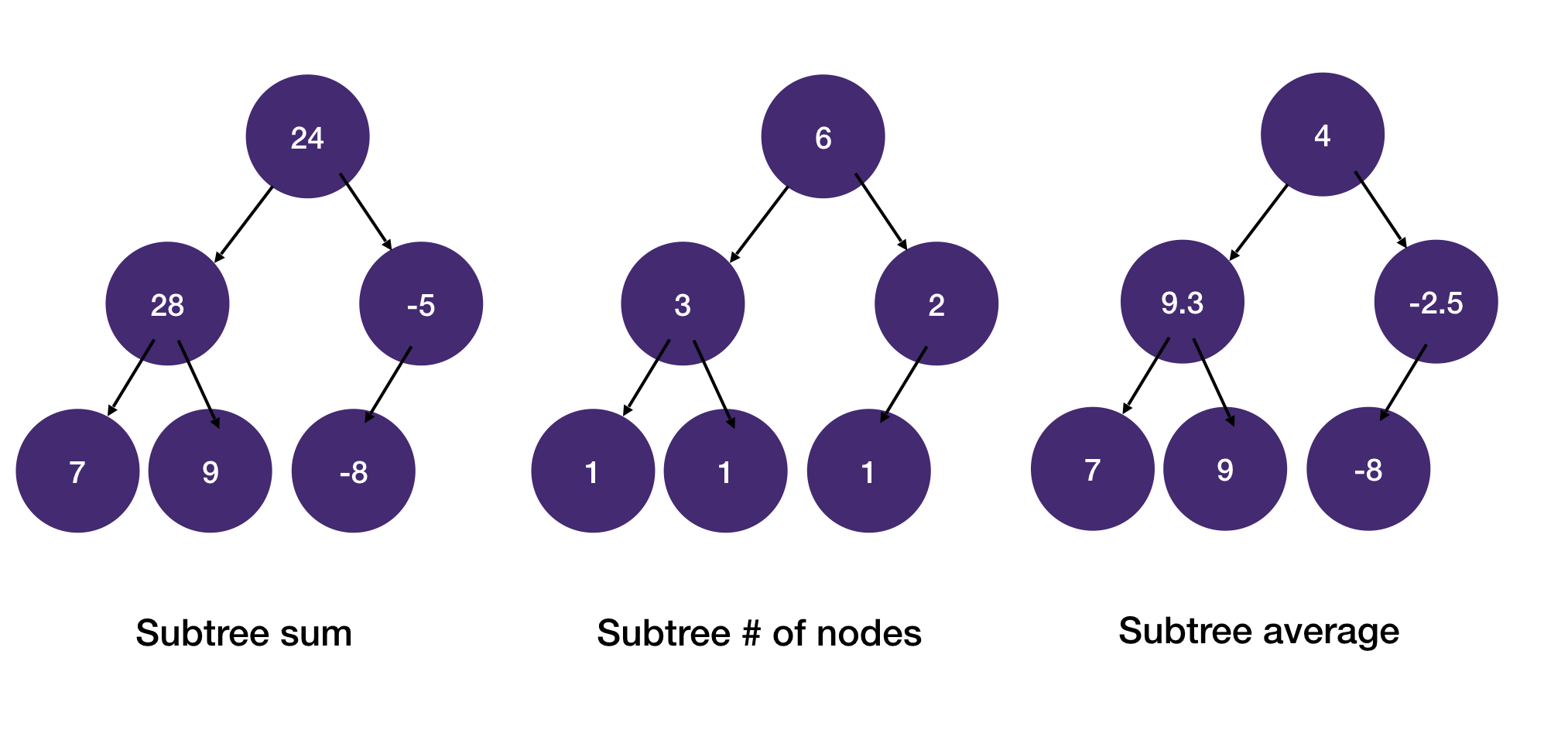Amazon Online Assessment (OA) - Subtree with Maximum Average

Given a tree, find the subtree with the maximum average value. Return the subtree's root's value. Note that the tree could have an arbitrary number of children.

Example 1:

Input:Explanation

The sum of each subtree:The subtree max average is 9.3, and the root of the subtree with max average is the node with value 12. So we return 12.

Explanation

Prereq: DFS on Tree, DFS on ternary tree

We traverse the tree with DFS and keep track of the sum of the subtree and the number of its children nodes.

1. Decide on the return value

The problem asks for the root node with the max average, so we update the max average value and its corresponding root node value as we go.

2. Identify states

To calculate the average of the subtree at each node, we need the subtree's sum and the number of children nodes. We can carry this as a state as we traverse the tree.

Having decided on the state and return value we can now write the DFS.

1from math import inf
2from typing import Tuple
3
4class Node:
5    def __init__(self, val, children=None):
6        if children is None:
7            children = []
8        self.val = val
9        self.children = children
10
11def subtree_max_avg(root: Node) -> int:
12    # (max_avg, root)
13    res = (-inf, None)
14
15    # -> (sum, num_nodes)
16    def dfs(node: Node) -> Tuple[int, int]:
17        nonlocal res
18        rec = [dfs(c) for c in node.children if c]
19        s = node.val + sum(t for t in rec)
20        n = 1 + sum(t for t in rec)
21        res = max(res, (s / n, node))
22        return s, n
23
24    dfs(root)
25    return res.val
26
27def build_tree(nodes, f):
28    val = next(nodes)
29    num = int(next(nodes))
30    children = [build_tree(nodes, f) for _ in range(num)]
31    return Node(f(val), children)
32
33if __name__ == '__main__':
34    root = build_tree(iter(input().split()), int)
35    res = subtree_max_avg(root)
36    print(res)
37